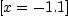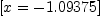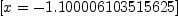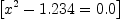login  home  contents  what's new  discussion  bug reports help  links  subscribe  changes  refresh  edit

 Submitted by : (unknown) at: 2007-11-17T23:03:38-08:00 (15 years ago) Name : Axiom Version : default friCAS-20090114 Axiom-20050901 OpenAxiom-20091012 OpenAxiom-20110220 OpenAxiom-Release-141 Category : Axiom Aldor Interface Axiom Compiler Axiom Library Axiom Interpreter Axiom Documentation Axiom User Interface building Axiom from source lisp system MathAction Doyen CD Reduce Axiom on Windows Axiom on Linux Severity : critical serious normal minor wishlist Status : open closed rejected not reproducible fix proposed fixed somewhere duplicate need more info Optional subject :   Optional comment :

[Kostas Oikonomou <opsl3ao4d515d6f0@mail.research.att.com> wrote:]

For anyone experienced with other symbolic mathematics systems, it is very difficult to understand why Axiom returns a complicated error message in this case!

axiom
solve(x+1.1,0.001)
There are 18 exposed and 3 unexposed library operations named solve
having 2 argument(s) but none was determined to be applicable.
Use HyperDoc Browse, or issue
)display op solve
package-calling the operation or using coercions on the arguments
will allow you to apply the operation.
Cannot find a definition or applicable library operation named solve
with argument type(s)
Polynomial(Float)
Float
Perhaps you should use "@" to indicate the required return type,
or "\$" to specify which version of the function you need.

[William Sit <wyscc@cunyvm.cuny.edu> replied:]

As with any software, one must learn the correct syntax of commands. For some unknown reason, Axiom does not have a solve function with signature:

solve: (Polynomial Float, Float) -> List Equation Polynomial Float. However, it has

solve: Polynomial Float->List Equation Fraction Polynomial Float

and solve: (Polynomial Fraction Integer, Float) -> List Equation Polynomial Float).

axiom
solve(x+1.1)(1)
Type: List(Equation(Fraction(Polynomial(Float))))
axiom
solve(x+11/10,0.1)(2)
Type: List(Equation(Polynomial(Float)))
axiom
solve(x+ 11/10,0.0001)(3)
Type: List(Equation(Polynomial(Float)))
axiom
solve(x+11/10,0.0000000000000000001)(4)
Type: List(Equation(Polynomial(Float)))

One should be careful interpreting these results. The second one solves it to 5 binary digit accuracy (closest binary to 0.1 (decimal) is 1/16+1/32 = 0.09375) hence the answer is not -1.1. A similar loss happens in the third one. To obtain -1.1 as the solution, one needs much higher accuracy, as in the fourth one (that is the minimum needed).

[[Kostas Oikonomou Sat Feb 12 09:03:08 -0600 2005 replied:]]

I'm confused. Perhaps that's because I expect Axiom to behave like other similar software, but what is the meaning of

solve: Polynomial Float->List Equation Fraction Polynomial Float

when we're trying to solve an equation with real coefficients? Doesn't an accuracy need to be specified? (and there doesn't seem to be a default).

[[William Sit replied:]]

Of course, yes. However, there is a dilemma: when you give Axiom an equation with floating point coefficients, should Axiom "solve" this symbolically, as if Float is just like any other domain, or numerically, giving Float a special treatment? Since Axiom algorithms are categorical, rather than writing two separate algorithms, Axiom solves, if possible, exactly and gives numerical answers as options when the precision parameter is given. This choice does not work well with equations over Float because Float does not have some of the algebraic properties as Fraction Integer or Fraction Complex Integer (such as factorization or GCD), which is why there is a warning in solve(x^2-1.234).

axiom
solve(x^2 - 1.234)
2
WARNING (genufact): No known algorithm to factor ?  - 1.234
, trying square-free.(5)
Type: List(Equation(Fraction(Polynomial(Float))))

The package is numsolve.spad and you see that these restrictions are well documented. So the above signature is really not meant to be used at the moment. A similar situation occurs, for example factor(1.23) is legal, but is really useless. Axiom does not use a mechanism to exclude specific domains from a category. It adopts an "include" philosophy but let things fail with warning or error. If you look into numsolve.spad, you will find that the innerSolve1 algorithm implementation is restricted. (So if later someone finds a way to implement a solve algorithm over Float, that would be just fine).

So a lot of Axiom failures are not bugs, but by design. One way to improve the user interface would seem to be to automatically lifting a polynomial over Float to one over Fraction Integer. A moment's reflection would convince you this is not always possible (for example, sqrt(2) or %pi technically both belong to Float (model for real numbers)), but of course, in reality, every (finite precision) floating point number is a rational number. Such a lifting package would have to take into consideration the precision to convert some symbolic constants to their decimal approximations and then convert them to exact rational numbers. However, even this would not create satisfactory results because we know the sensitivity of solutions of polynomial equations to small changes of its coefficients. Wilkinson has this example

f(x) = (x+1)(x+2) ... (x+20) = x20 + 210 x19 + ... + 20! = 0

where a change of the coefficient 210 by 2−23 (approximately 1.2 × 10−7) would turn the root −20 to −20.8 and five pairs of zeros to complex roots. (This perturbed equation will take a very long time in Axiom, will not be solved exactly by Mathematica, but is easily solved numerically in Mathematica).

So if we want numerically accurate solutions, we should use a robust numerical library. I believe this is not yet available in Axiom (the NAG version allowed interface with its Fortran libraries, at extra costs).

If we are really (no pun intended) only using truely floating point coefficients, then it can easily be converted to Fraction Integer, but one has to beware that the algorithm would take a very long time because exact arithmetic with large integer coefficients are expensive.

[[Kostas Oikonomou wrote:]]

Also, while solve(x+1.1) "works", so to speak, solve(x^2 - 1.234) returns a warning.

Very bewildering to a beginning user!

My impression, from looking at the algebra library, is that Axiom does not appear to handle (i.e. solve) polynomials with real coefficients. It produces them as results, but doesn't accept them as inputs. Is this basically correct?

[[Martin Rubey Sat Feb 12 13:07:55 -0600 2005 <martin.rubey@univie.ac.at> wrote:]]

I think the idea is to convert your Polynomial Float into a Polynomial Fraction Integer and then use solve (POLY FRAC INT, FLOAT) -> ...  to get results.

[[William Sit wrote:]]

Yes, as explained above. Symbolic methods do not work well with Float.

property change --Bill Page, Wed, 18 May 2005 06:54:00 -0500 reply
Category: MathAction => Axiom Interpreter

 Subject:   Be Bold !! ( 14 subscribers )